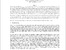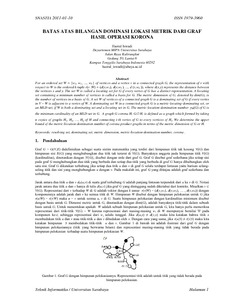# Batas Atas Bilangan Dominasi Lokasi Metrik Graf Hasil Operasi Korona

Iswadi, Hazrul (2011) Batas Atas Bilangan Dominasi Lokasi Metrik Graf Hasil Operasi Korona. Prosiding Seminar Nasional Teknologi Informasi dan Multimedia 2011 (SNASTIA 2011). pp. 1-5. ISSN 1979-3960Preview PDF hazrul_Batas Bilangan Dominasi Lokasi Metrik_2011.pdf - Published Version Download (162kB) | Preview

## Abstract

For an ordered set W = {w_1, w_2 , ..., w_k } of vertices and a vertex v in a connected graph G, the representation of v with respect to W is the ordered k-tuple r(v |W) = (d(v,w_1), d(v,w_2 ), ..., d (v,w_k )), where d(x,y) represents the distance between the vertices x and y. The set W is called a locating set for G if every vertex of G has a distinct representation. A locating set containing a minimum number of vertices is called a basis for G. The metric dimension of G, denoted by dim(G), is the number of vertices in a basis of G. A set W of vertices of a connected graph G is a dominating set of G if every vertex in V – W is adjacent to a vertex of W. A dominating set W in a connected graph G is a metric-locating-dominating set, or an MLD-set, if W is both a dominating set and a locating set in G. The metric-location-domination number \gamma_M(G) of G is the minimum cardinality of an MLD-set in G. A graph G corona H, G \odot H, is defined as a graph which formed by taking n copies of graphs H_1, H_2, …, H_n of H and connecting i-th vertex of G to every vertices of Hi. We determine the upper bound of the metric-location-domination number of corona product graphs in terms of the metric dimension of G or H.

Item Type: Article resolving set, dominating set, metric dimension, metric-location-domination number, corona Q Science > QA Mathematics Academic Department > Department of Mathematics and Natural Science Hazrul Iswadi 6179 29 Mar 2012 05:37 24 Mar 2021 14:17 http://repository.ubaya.ac.id/id/eprint/272View Item